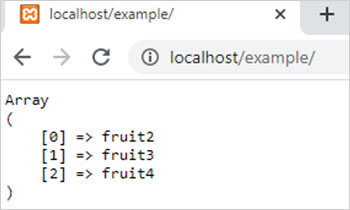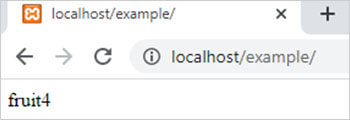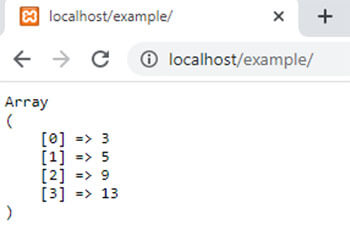array_rand() function in PHP || PHP Arrays# array_rand() function in PHP

1604

array_rand() function of PHP is used to return one or more random keys from an array where the number of keys defined by the user. If the number of keys return is more than one then it returns an array of keys. It is an inbuilt function of PHP.

Syntax:

array_rand(\$array,\$no_of_keys);

here,

\$array is a PHP array and \$no_of_keys is the number of keys which we want to return.

Example 1:

```<?php
\$arr=array("fruit1"=>"Apple","fruit2"=>"Mango","fruit3"=>"Grapes","fruit4"=>"pear","fruit5"=>"orange","fruit6"=>"banana");
\$result=array_rand(\$arr,3);
echo "<pre>";
print_r(\$result);
?>```

Output:Example 2:

```<?php
\$arr=array("fruit1"=>"Apple","fruit2"=>"Mango","fruit3"=>"Grapes","fruit4"=>"pear","fruit5"=>"orange","fruit6"=>"banana");
\$result=array_rand(\$arr,1);
echo \$result;
?>```

Output:Example 3:

```<?php
\$arr=array(1,2,3,4,5,6,7,8,9,10,11,12,13,14,15);
\$result=array_rand(\$arr,4);
echo "<pre>";
print_r(\$result);
?>```

Output:Share:## Big Hosting Days

Upto 75@ OFF on Web Hosting

## Get a .COM for just \$6.98

Secure Domain for a Mini Price